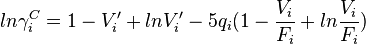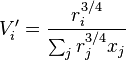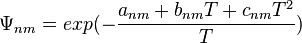# Modified UNIFAC (Dortmund)

The following modifications are made to the Original UNIFAC model:

Combinatorial part of liquid activity coefficient:$ln \gamma^C_i = 1 - V'_i + ln V'_i - 5 q_i (1 - \frac{V_i}{F_i} + ln \frac{V_i}{F_i})$

Volume/mole fraction ratio:$V_i' = \frac{r_i^{3/4}}{\sum_j r_j^{3/4} x_j}$

Main group interaction parameter matrix:$\Psi_{nm} = exp(- { {a_{nm} + b_{nm} T + c_{nm} T^2} \over {T} })$